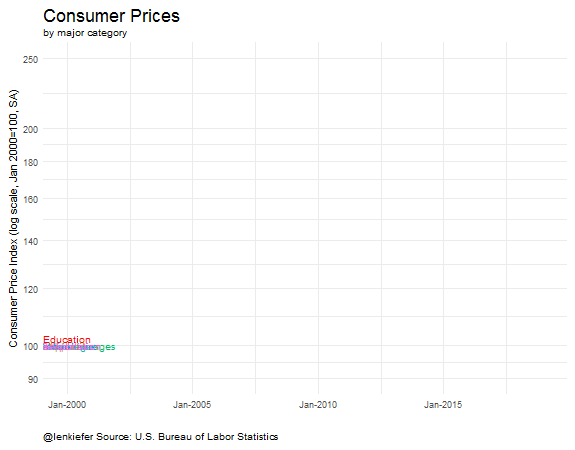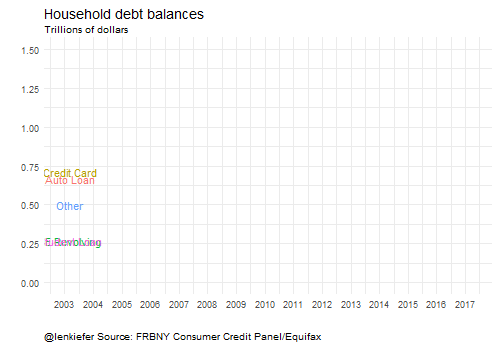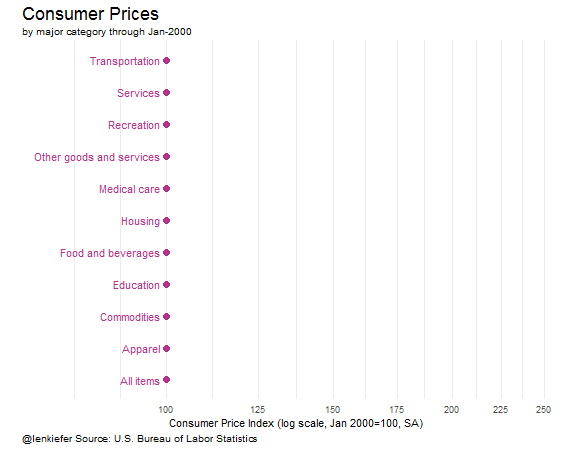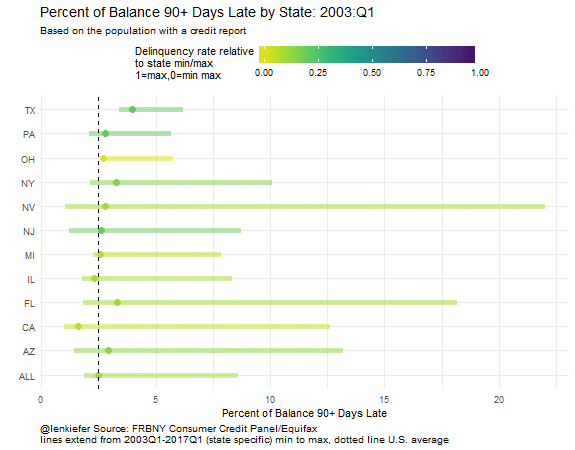# Consumer prices, household debt

LET’S TAKE A LOOK AT RECENT TRENDS IN CONSUMER PRICES AND HOUSEHOLD DEBT. Along the way we’ll refresh some visualizations of consumer prices (see here) and household debt (see here) we made last year, as well as think up some new ones.

As usual we’ll use R to generate the plots and I’ll share the code below. But before we get into the details of constructing the charts, let’s just look at two plots to help set the stage.

# Step 1: get the data

The data for these plots are conveniently made available by the BLS and the New York Fed.

A quick note on data manipulation. I have been cycling between using a data.table based approach for data wrangling and a dplyr/tidyverse based approach. Both seem to work. For the CPI data I’ll mostly use data.table and for the household debt data I’ll mostly use dplyr.

## Consumer price data

First, we’ll follow my CPI post to get the Consumer Price data.

###############################################################################
###############################################################################
library(data.table,quietly=T,warn.conflicts=F)
library(tidyverse,quietly=T,warn.conflicts=F)

###############################################################################
#### Read in data from BLS
###############################################################################

# get data

# Get item names
"selectable","sort.sequence","blank"))
# get series info
col.names=c("series_id","area_code","item_code","seasonal",
"periodicity_code","base_code","base_period",
"footnote_codes","begin_year","begin_period",
# merge
cpi2<-merge(cpi.item,cpi.series,by.x="item.code",by.y="item_code")

setkeyv(cpi1,"series_id")
setkeyv(cpi2,"series_id")
cpi3<-cpi2[cpi1]
# create date
cpi3<-cpi3[,month:=as.numeric(substr(cpi3$period,2,3))] cpi3$date<- as.Date(ISOdate(cpi3$year,cpi3$month,1) )

# subset data
cpi4<-cpi3[area_code=="0000" & seasonal=="S" & item.code!= "SAA1" & item.code !="SAA2"]
# get January 2000 data
bdata<-cpi4[year==2000 & month==1,]
bdata<-dplyr::rename(bdata, value00=value)
bdata<-bdata[, c('value00','series_id'), with = FALSE]
cpi5<-merge(cpi4,bdata,by="series_id")

# reindex data so that 2000 = 100
cpi5<-cpi5[,cpi00:=100*value/value00]
cpi6<-cpi5[year>1999]
xlim<-c(min(cpi6$date),max(cpi6$date))
dd<-unique(cpi6$date) # Originally, had Education combined with communication (as BLS reports it) # let's just get education # Series id: CUSR0000SAE1 cpi.ed<-fread("http://download.bls.gov/pub/time.series/cu/cu.data.17.USEducationAndCommunication") cpi.ed[,month:=as.numeric(substr(cpi.ed$period,2,3))]
cpi.ed$date<- as.Date(ISOdate(cpi.ed$year,cpi.ed$month,1) ) cpi.ed$item.name<-"Education"
cpi.ed<-cpi.ed[series_id=="CUSR0000SAE1"]
bdata.ed<-cpi.ed[year==2000 & month==1,]
bdata.ed<-dplyr::rename(bdata.ed, value00=value)
bdata.ed<-bdata.ed[, c('value00','series_id'), with = FALSE]
cpi.ed<-merge(cpi.ed,bdata.ed,by="series_id")
cpi.ed[,cpi00:=100*value/value00]

## Household debt data

The household debt data is available in an Excel spreadsheet. We’ll have to use readxl (see this post for more on wrangling Excel data with R). Let’s start by assuming that you have downloaded the latest credit panel summary data (available here .xlsx file) and saved it as HHD_C_Report_2017Q1.xlsx in a data directory.

Now in order to get this data useable, we have to use readxl to reach into the files and extract the useful information. I had originally been doing some manual manipulation in Excel, but ewww gross, let’s try to do it all with R.

It’s going to involve understanding the structure of the Excel file and using some of readxl’s nice features.

This spreadsheet is-sigh-well, set up like spreadsheets are wont to be. The data we want is in the “Page 3 Data” sheet, organized with variables in rows and data in columns. The row titles we want are not contiguous with the data (there’s a gap of a couple columns). We’ll have to import the data and try to beat it into tidy submission so we can actually work with it.

# We'll use the tidyverse here
library(tidyr,quietly=T,warn.conflicts=F)
library(dplyr,quietly=T,warn.conflicts=F)
library(purrr,quietly=T,warn.conflicts=F)

# data is range D4: BH10, we'll get the data values from row 3
# the row titles are in column A, so we'll get it and then delete blanks

sheet = "Page 3 Data",  # name of sheet
range="A3:BH10" )        # range where data lives

## we've got blank columns in positions 2 & 3 (drop them)
## then we'll gather the data and rename X__1 as "type"

df2<-df %>% select(-X__2,-X__3) %>% gather(datec,debt,c(2:58)) %>% rename(type=X__1)

#now create a date variable using the datec string:

df2<- df2 %>%
mutate(year=2000+as.numeric(substr(datec,1,2)),
month=as.numeric(substr(datec,5,5))*3) %>%
# create date variable
mutate(date=as.Date(ISOdate(year,month,1))) %>%
# drop unneeede variables
select(type,date,debt)

Hey, not so bad. Let’s make a table looking at the last quarter of data (2017 Q1).

# Make a table for 2017 Q1:

htmlTable(
df2 %>% filter(date=="2017-03-01") %>%
arrange(-debt) %>%
map_if(is.numeric,scales::dollar) %>% as.data.frame(),
col.rgroup = c("none", "#F7F7F7"),
caption="Aggregate Household Debt ($Trillions, 2017 Q1)", tfoot="Source: FRBNY Consumer Credit Panel/Equifax")  type date debt Aggregate Household Debt ($ Trillions, 2017 Q1) 1 Total 2017-03-01 $12.72 2 Mortgage 2017-03-01$8.63 3 Student Loan 2017-03-01 $1.34 4 Auto Loan 2017-03-01$1.17 5 Credit Card 2017-03-01 $0.76 6 HE Revolving 2017-03-01$0.46 7 Other 2017-03-01 $0.37 Source: FRBNY Consumer Credit Panel/Equifax # Step 2: make some awesome charts Now that we’ve got our data, we can start making some awesome charts. Or maybe I should say continue making awesome charts, because we never really stop do we? Let’s get to it. ## Venerable line charts We’ll get crazy I promise but let’s start simple with the venerable line chart. The code below will recreate the plots we started with. ### CPI line chart library(ggrepel,quietly=T,warn.conflicts=F) # for the labels (avoid overplotting) # dd is a list of dates, we'll set i = max date for labels xlim<-c(min(cpi6$date),max(cpi6$date)) dd<-unique(cpi6$date)
i<- length(dd)

ggplot(data=cpi6[
#drop some items for clarity
! (item.name %in% c("Other goods and services","Services",
"Education and communication"))],
aes(x=date,y=cpi00,color=item.name))+
geom_line()+
theme_minimal()+   theme(legend.justification=c(0,0), legend.position="none")+
scale_y_log10(limits=c(90,250),breaks=c(90,100,120,140,160,180,200,250))+
geom_line(data=cpi.ed[ year>1999],size=1.5,color="red")+
scale_x_date(labels= date_format("%b-%Y"),
limits = as.Date(c('2000-01-01','2018-12-31')))+
geom_text_repel(
data = cpi6[date==dd[i] &
! (item.name %in% c("Other goods and services","Services",
"Education and communication"))],
aes(label = item.name), size = 3.5, nudge_x = 1) +
geom_text(
data = cpi.ed[date==dd[i]],color="red",
aes(label = item.name),
size = 3.5,nudge_x = 1,nudge_y=.01) +
labs(x="", y="Consumer Price Index (log scale, Jan 2000=100, SA)",
title="Consumer Prices",
subtitle="by major category",
caption="@lenkiefer Source: U.S. Bureau of Labor Statistics")+
theme(plot.title=element_text(size=18),
plot.caption=element_text(hjust=0,vjust=1),
plot.margin=unit(c(0.25,0.25,0.25,0.25),"cm"))### Household debt line chart

ggplot(data=filter(df2,type!="Total" & type !="Mortgage"),
aes(x=date,y=debt,color=type,label=type))+
geom_line(size=1.2)+theme_minimal()+
geom_text(data=tail(filter(df2,type!="Total" & type !="Mortgage"),5),
nudge_x=10,nudge_y=.025)+
coord_cartesian(xlim=c(as.Date("2003-01-01"),as.Date("2017-12-31")), y=c(0,1.5))+
theme(plot.title=element_text(size=14),
plot.caption=element_text(hjust=0,vjust=1),
legend.position="none")+
labs(title="Household debt",subtitle="Trillions of dollars",x="",y="",
caption="@lenkiefer Source: FRBNY Consumer Credit Panel/Equifax")### Inflation line chart

The CPI line chart compares price changes over a long horizon. Let’s focus on more recent changes. The code below generates a plot of monthly price inflation by category.

# stack education with full data
cpi7<-rbind(cpi6,cpi.ed,fill=T)

# construct monthly inflation metrics
cpi7 <- cpi7[order(series_id,date),]
cpi7<-cpi7[, cpi.inf:= value/shift(value,1,fill=NA)-1, by=series_id]

# plot data
ggplot(data=cpi7[
#drop some items for clarity
! (item.name %in% c("Other goods and services","Services",
"Education and communication"))],
aes(x=date,y=cpi.inf,color=item.name))+
geom_line()+
geom_hline(yintercept=0,linetype=2,color="darkgray")+
scale_y_continuous(label=scales::percent)+
theme_minimal()+   theme(legend.justification=c(0,0), legend.position="none")+
scale_x_date(labels= date_format("%Y"),
limits = as.Date(c('2000-01-01','2017-12-31')))+
geom_text_repel(
data = cpi7[date==dd[i] &
! (item.name %in% c("Other goods and services","Services",
"Education and communication"))],
aes(label = item.name), size = 3.5, nudge_x = 1) +
labs(x="", y="Consumer Price Inflation (Monthly % change, SA)",
title="Consumer Price Inflation (m/m %)",
subtitle="by major category",
caption="@lenkiefer Source: U.S. Bureau of Labor Statistics")+
theme(plot.title=element_text(size=18),
plot.caption=element_text(hjust=0,vjust=1),
plot.margin=unit(c(0.25,0.25,0.25,0.25),"cm"))Wow, that’s pretty ugly, transportation is volatile and it’s hard to see what’s going on in the plot. Let’s use faceting to make a small multiple (also, let’s drop volatile transportation/commodities and recreation so we have 6 categories). Let’s also zoom in on trends since 2010.

# plot data
ggplot(data=cpi7[
#drop some items for clarity
! (item.name %in% c("Other goods and services","Services",
"Education and communication", "Transportation",
"Commodities","Recreation"))],
aes(x=date,y=cpi.inf,color=item.name))+
geom_line()+
geom_hline(yintercept=0,linetype=2,color="darkgray")+
scale_y_continuous(label=scales::percent)+
theme_minimal()+   theme(legend.justification=c(0,0), legend.position="none")+
scale_x_date(labels= date_format("%Y"),
limits = as.Date(c('2010-01-01','2017-12-31')))+
facet_wrap(~item.name)+
labs(x="", y="Consumer Price Inflation (monthly % change, SA)",
title="Consumer Price Inflation (m/m %)",
subtitle="by major category",
caption="@lenkiefer Source: U.S. Bureau of Labor Statistics")+
theme(plot.title=element_text(size=18),
plot.caption=element_text(hjust=0,vjust=1),
plot.margin=unit(c(0.25,0.25,0.25,0.25),"cm"))Getting better. Still quite a bit of noise in some of the monthly measures. Let’s try looking at year-over-year percent changes to smooth out some of that noise.

# get 12 month inflation
cpi7<-cpi7[, cpi.inf12:= value/shift(value,12,fill=NA)-1, by=series_id]

# plot data
ggplot(data=cpi7[
#drop some items for clarity
! (item.name %in% c("Other goods and services","Services",
"Education and communication", "Transportation",
"Commodities","Recreation"))],
aes(x=date,y=cpi.inf12,color=item.name))+
geom_line()+
geom_hline(yintercept=0,linetype=2,color="darkgray")+
scale_y_continuous(label=scales::percent)+
theme_minimal()+   theme(legend.justification=c(0,0), legend.position="none")+
scale_x_date(labels= date_format("%Y"),
limits = as.Date(c('2010-01-01','2017-12-31')))+
facet_wrap(~item.name)+
labs(x="", y="Consumer Price Inflation (12-month % change, SA)",
title="Consumer Price Inflation (y/y %)",
subtitle="by major category",
caption="@lenkiefer Source: U.S. Bureau of Labor Statistics")+
theme(plot.title=element_text(size=18),
plot.caption=element_text(hjust=0,vjust=1),
plot.margin=unit(c(0.25,0.25,0.25,0.25),"cm"))Now we have a clearer picture of recent trends. Overall inflation has been rising over the past year or so (thanks largely to recovering energy prices). Both Medical Care and Education are rising at a level well above general inflation, but seem to be trending down. Housing, which measures rents and owner’s equivalent rent not house prices (see for example question 2 here), is on the upswing.

## Horizon charts

Let’s build some horizon charts (see my horizon chart post for everything you want, everything you need) to analyze inflation trends. Making horizon charts was only possible due to code from [@timelyportfolio](https://twitter.com/timelyportfolio), lots of cool stuff over at his page.

# subset data
df.cpi<-cpi7[ year(date)>2009 &  !
(item.name %in% c("Other goods and services",
"Services","Education and communication",
"Transportation","Recreation")), c("date","item.name","cpi.inf12"),
with=F]
############# magic! ##############################################################

library(htmltools)
library(DT)
library(d3horizonR)

myf3<-function (s="Ohio"){
d.out<- filter(df.cpi,item.name==s)$cpi.inf12 return(d.out) } dt3 <- data.frame(item.name=unique(df.cpi$item.name))

dt4 <- dt3 %>%
mutate(x = lapply(item.name, myf3 ) )  %>%
mutate(x = lapply(x, function(dat) {
d3horizon_chr(
list(dat),
options = d3horizonOptions(height=20),
width = 400
)
}) )

m<-
datatable(
dt4,
caption = 'Annual growth in consumer prices',
escape = FALSE,
colnames=c("Item","12-month % change\n in prices"),
options = list(
columnDefs = list(list(width="400px", targets = 2:2)),
fnDrawCallback = htmlwidgets::JS()
)
) %>%
tagList(htmlwidgets::getDependency("d3horizon", "d3horizonR")) %>%
browsable()

# save m
library(htmlwidgets)
saveWidget(m, file="m.html")

These widgets are cool, but I’m not doing much with them here. We’ll do more later.

## Lollipops

Lollipop charts have been under fire recently, but I still like them. See here for a more spirited defense of the chart type from Andy Cotgreave.

We’ll just go ahead and make one. If you don’t like them, the nice thing about ggplot2 is it’s pretty easy to just swap in a bar.

Let’s make one.

library(viridis) # for the colors!
ggplot(data=cpi7[date==dd[i] & item.name != "Education and communication",],
aes(xend=100,x=cpi00,
yend=reorder(item.name,cpi00),y=reorder(item.name,cpi00),
color=cpi00,
label=paste(" ",item.name," ")))+
geom_segment(size=0.25,linetype=2)+geom_point(size=3)+
scale_x_log10(limits=c(75,250),breaks=seq(100,250,25))+
theme_minimal()+
geom_text(aes(hjust=ifelse(cpi00>100,0,1)))+
scale_color_viridis(name="Price Index",option="C",end=0.85)+
labs(y="", x="Consumer Price Index (log scale, Jan 2000=100, SA)",
title="Consumer Prices",
subtitle=paste("by major category through",as.character(dd[i],format="%b-%Y")),
caption="@lenkiefer Source: U.S. Bureau of Labor Statistics")+
theme(plot.title=element_text(size=18),
legend.position="none",
axis.text.y=element_blank(),
panel.grid.major.y=element_blank(),
panel.grid.minor.y=element_blank(),
plot.caption=element_text(hjust=0))This plot compares how much prices have risen relative to 2000. Essentially, this is just the endpoint of the line plot.

# Movie versions

These data lend themself to animations.

Here’s some animated gifs of some of the plots:# Wrapping up

Time to wrap up for today. We refreshed some old charts and introduced a couple new ones. We did some data wrangling with data.table() and dplyr. We used readxl to reach into Excel files and get our data. And we tried out forcats to reorder labels in our chart. All this enabled us wrangle our data and get it ready to use ggplot2 to visualize trends in consumer prices and household debt.

Hope you found this useful. How could these code snippets and data visualizations work for you?